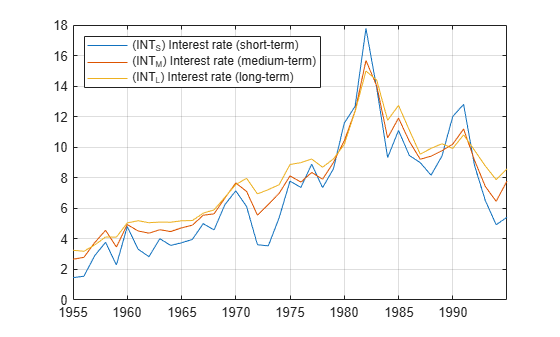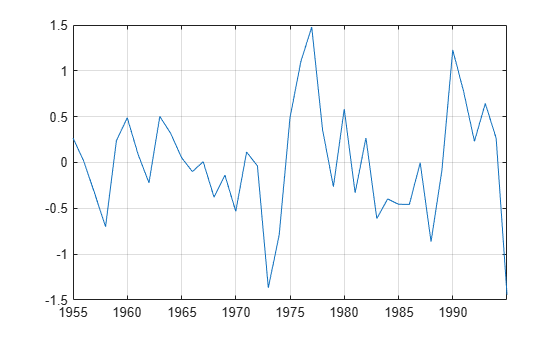egcitest

Engle-Granger cointegration test

Syntax

[h,pValue,stat,cValue,reg1,reg2] = egcitest(Y)
[h,pValue,stat,cValue,reg1,reg2] = egcitest(Y,Name,Value)

Description

Engle-Granger tests assess the null hypothesis of no cointegration among the time series in Y. The test regresses Y(:,1) on Y(:,2:end), then tests the residuals for a unit root.

[h,pValue,stat,cValue,reg1,reg2] = egcitest(Y) performs the Engle-Granger test on a data matrix Y.

[h,pValue,stat,cValue,reg1,reg2] = egcitest(Y,Name,Value) performs the Engle-Granger test on a data matrix Y with additional options specified by one or more Name,Value pair arguments.

Input Arguments

 Y numObs-by-numDims matrix representing numObs observations of a numDims-dimensional time series y(t), with the last observation the most recent. Y cannot have more than 12 columns. Observations containing NaN values are removed.

Name-Value Arguments

Specify optional comma-separated pairs of Name,Value arguments. Name is the argument name and Value is the corresponding value. Name must appear inside quotes. You can specify several name and value pair arguments in any order as Name1,Value1,...,NameN,ValueN.

 creg Character vector, such as 'nc', or cell vector of character vectors indicating the form of the cointegrating regression, where y1 = Y(:,1) is regressed on Y2 = Y(:,2:end) and optional deterministic terms in X:y1 = Xa + Y2b + εValues are: 'nc'—no constant or trend in X'c'—constant but no trend in X'ct' —constant and linear trend in X'ctt' —constant, linear trend, and quadratic trend in X Default: 'c' cvec Vector or cell vector of vectors containing coefficients [a;b] to be held fixed in the cointegrating regression. The length of a is 0, 1, 2 or 3, depending on creg, with coefficient order: constant, linear trend, quadratic trend. The length of b is numDims − 1. It is assumed that the coefficient of y1 = Y(:,1) has been normalized to 1. NaN values indicate coefficients to be estimated. If cvec is completely specified (no NaN values), no cointegrating regression is performed. Default: Completely unspecified cointegrating vector (all NaN values). rreg Character vector, such as 'ADF', or cell vector of character vectors indicating the form of the residual regression. Values are: 'ADF' — augmented Dickey-Fuller test of residuals from the cointegrating regression'PP' — Phillips-Perron test Test statistics are computed by calling adftest and pptest with the model parameter set to 'AR', assuming data have been demeaned or detrended, as necessary, in the cointegrating regression. Default: 'ADF' lags Scalar or vector of nonnegative integers indicating the number of lags used in the residual regression. The meaning of the parameter depends on the value of rreg (see the documentation for the lags parameter in adftest and pptest). Default: 0 test Character vector, such as 't1', or cell vector of character vectors indicating the type of test statistic computed from the residual regression. Values are: 't1' — a “τ test”'t2' — a “z test” The meaning of the parameter depends on the value of rreg (see the documentation for the test parameter in adftest and pptest). Default: t1 alpha Scalar or vector of nominal significance levels for the tests. Values must be between 0.001 and 0.999. Default: 0.05

Single-element parameter values are expanded to the length of any vector value (the number of tests). Vector values must have equal length. If any value is a row vector, all outputs are row vectors.

Output Arguments

h

Vector of Boolean decisions for the tests, with length equal to the number of tests. Values of h equal to 1 (true) indicate rejection of the null in favor of the alternative of cointegration. Values of h equal to 0 (false) indicate a failure to reject the null.

pValue

Vector of p-values of the test statistics, with length equal to the number of tests. p-values are left-tail probabilities.

stat

Vector of test statistics, with length equal to the number of tests. The statistic depends on the rreg and test values (see the documentation for adftest and pptest).

cValue

Vector of critical values for the tests, with length equal to the number of tests. Values are for left-tail probabilities. Since residuals are estimated rather than observed, critical values are different from those used in adftest or pptest (unless the cointegrating vector is completely specified by cvec). egcitest loads tables of critical values from the file Data_EGCITest.mat, then linearly interpolates test values from the tables. Critical values in the tables were computed using methods described in .

reg1

Structure of regression statistics from the cointegrating regression.

reg2

Structure of regression statistics from the residual regression.

The number of records in reg1 and reg2 equals the number of tests. Each record has the following fields:

 num Length of the regression response y, with NaNs removed size Effective sample size, adjusted for lags, difference* names Regression coefficient names coeff Estimated coefficient values se Estimated coefficient standard errors Cov Estimated coefficient covariance matrix tStats t statistics of coefficients and p-values FStat F statistic and p-value yMu Mean of y, adjusted for lags, difference* ySigma Standard deviation of y, adjusted for lags, difference* yHat Fitted values of y, adjusted for lags, difference* res Regression residuals DWStat Durbin-Watson statistic SSR Regression sum of squares SSE Error sum of squares SST Total sum of squares MSE Mean squared error RMSE Standard error of the regression RSq R2 statistic aRSq Adjusted R2 statistic LL Loglikelihood of data under Gaussian innovations AIC Akaike information criterion BIC Bayesian (Schwarz) information criterion HQC Hannan-Quinn information criterion

*Lagging and differencing a time series reduces the sample size. Absent any presample values, if y(t) is defined for t = 1:N, then the lagged series y(tk) is defined for t = k+1:N. Differencing reduces the time base to k+2:N. With p lagged differences, the common time base is p+2:N and the effective sample size is N−(p+1).

Examples

collapse all

Y = Data(:,3:end);
names = series(3:end);
plot(dates,Y)
legend(names,'location','NW')
grid onTest for cointegration (and reproduce row 1 of Table II in ).

[h,pValue,stat,cValue,reg] = egcitest(Y,'test',...
{'t1','t2'});
h,pValue
h = 1x2 logical array

0   1

pValue = 1×2

0.0526    0.0202

Plot the estimated cointegrating relation ${\mathit{y}}_{1}-{\mathit{Y}}_{2}\mathit{b}-\mathrm{Xa}$.

a = reg(2).coeff(1);
b = reg(2).coeff(2:3);
plot(dates,Y*[1;-b]-a)
grid onAlgorithms

A suitable value for lags must be determined in order to draw valid inferences from the test. See notes on the lags parameter in the documentation for adftest and pptest.

Samples with less than ~20 to 40 observations (depending on the dimension of the data) can yield unreliable critical values, and so unreliable inferences. See .

If cointegration is inferred, residuals from the reg1 output can be used as data for the error-correction term in a VEC representation of y(t). See . Estimation of autoregressive model components can then be performed with estimate, treating the residual series as exogenous.

References

 Engle, R. F. and C. W. J. Granger. “Co-Integration and Error-Correction: Representation, Estimation, and Testing.” Econometrica. v. 55, 1987, pp. 251–276.

 Hamilton, J. D. Time Series Analysis. Princeton, NJ: Princeton University Press, 1994.

 MacKinnon, J. G. “Numerical Distribution Functions for Unit Root and Cointegration Tests.” Journal of Applied Econometrics. v. 11, 1996, pp. 601–618.